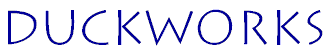NEW HOME INPUT INDEXES FEATURES CLASSIFIED CONTESTS ARCHIVES FORUM STORE LINKS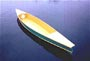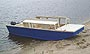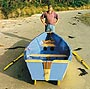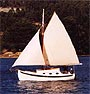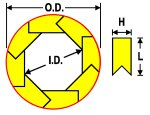Bird's Mouth Spars, The Appendix
 Contents The formulas used for analysis Data Tables

Formulas

In this section, the formulas used for this article will be shown. These are excerpts from my personal notes I wrote down in order to prepare this article. Since these notes covered a dozen of pages or so in Word format, I will try to shorten this as much as possible. Most equations have been numbered for reference purpose. But not all will necessarily be presented. So some equation numbers may be missing, while other will appear out of sequence.

Size Calculations

These are the original Farless equations for calculating size:

 O.D. = 2R R = R1 + H R1 = R2 cos ½ a I.D. = 2 R2L1 = ½ (L - H cos a) a = 360° / N, where N is the number of sides

The formula for L1 needs to be adjusted to work for any number of sides. For an 8-sided spar, the V-notch uses equal 45-degree angles. At 45 degrees, the sine and cosine have the same value. So in this particular case, the formula will work with either the sine or cosine function. For all possible configurations, we have to use the sine function for the formula to work:

 L1 = ½ (L - H sin a) revised equation

All the other formulas stay the same. By combining all the "intermediate" values (R1, R2 and L1), a few simplifications were possible. The final revised equation for calculating O.D. is: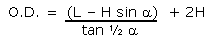Equation 1

And the final revised equation for I.D. is:Equation 2A

By replacing "H" with "K", these equations become:Equation 2B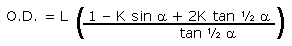Equation 4A

From these last two equations, we can extract a lot of useful information. The long section between brackets in Equation 4A is in fact the value for ratio M: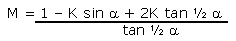Equation 22

Here is the formula for calculating ratio K from ratio A: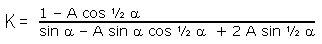Equation 21A

For a round exterior, the theoretical minimum and maximum limits for the staves' thickness ratio K are:Equation 19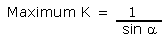Equation 20

For a polygon exterior, the minimum K is zero!

One side note, here: below is an alternate equation for size I had figured out on my own. But it only works for an 8-sided spar. For any other configuration, it would have to be scrapped and re-written from scratch. The approach used in the Farless equations is more universal. The value for the angle (alpha), in the revised equations presented here, automatically handles a different number of sides. That's why this alternate equation is now obsolete.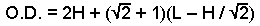Obsolete alternate equation: for 8-sided spars only

Weight saving compared to a solid spar of the same size

These formulas are more accurate than the first approximation presented in the previous article by David Farless. But they are substantially more complex. The first approximation, in terms of weight saving, was:

Weight Saving (%) = 100 ( I.D. /O.D.) 2

In order to compare spars made up of a different number of sides, the weight saving must be defined as:

Weight Saving (%) = 100 ( Empty Area / Solid Round Area )

The complete formula that fits this definition is:Equation 9B

The definition and formula for a polygon exterior are actually more complex than a round exterior:

The relative mass for a hollow polygon, compared to a solid (included) circle is:

Relative Mass = ( Exterior Polygon Area - Empty Area ) / Circle Area

The weight saving (in percent) is:

Weight Saving (Polygon)(%) = 100 ( 1 - Relative Mass)

And the complete formula is: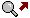(Click to enlarge) Equation 10

Strength Loss

For a round exterior spar, the strength loss is defined as:

Strength Loss (%) = 100 ( I.D. / O.D. ) 4

Using the values of I.D and O.D. defined in equations 2B and 4A we obtain the following formula: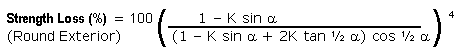Equation 11

For a polygon outside shape, the outside diameter used is the minimum value. The minimum outside diameter is the same value as O.D. for a round exterior. Since the ratio (I.D./O.D.) is constant all around, the worst case figure is calculated using the minimum I.D. as well. The minimum inside diameter is twice R1 (see Figure 1).

Strength Loss (%) = 100 ( minimum I.D. / O.D. ) 4 = 100 ( I.D. cos ½ a / O.D. ) 4

Strength Loss (%) = 100 ( A cos ½ a ) 4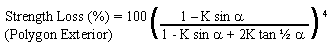Equation 12

Size increase required for same strength as solid spar (Round Exterior)

This is kind of the inverse of the strength loss formula.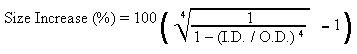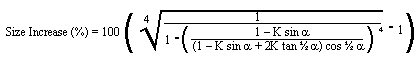(Click to enlarge) Equation 15

Size increase required for same strength as solid spar (Polygon Exterior)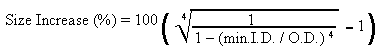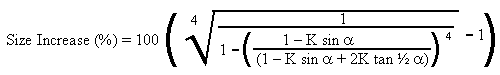Equation 16

Weight Saving for the bigger hollow spar (Round Exterior)

This is the weight saving equation for a bird's mouth spar having a strength equivalent to a solid round spar. The next two equations would just be too long to show in their fully developed form.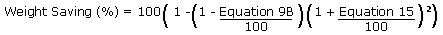Equation 17

Weight saving for the bigger hollow spar (Polygon Exterior)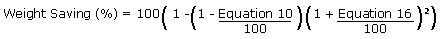Equation 18

Outside Waste

The next two equations fall in the category: "nice to know but way too much trouble to use!" The definitions are understandable, but the equations needed to fit the definitions are so long, they have to be broken in segments to work in a spreadsheet! The outside waste that must be removed for a round bird's mouth spar is defined as: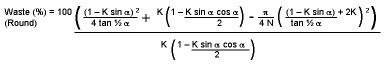(Click to enlarge) Equation 13

The outside waste to be removed for a polygon exterior is defined as: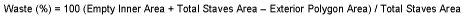And the formula for this is:(Click to enlarge) Equation 14